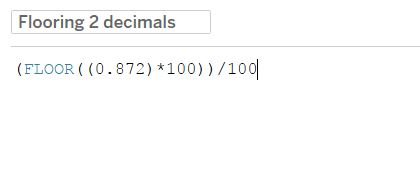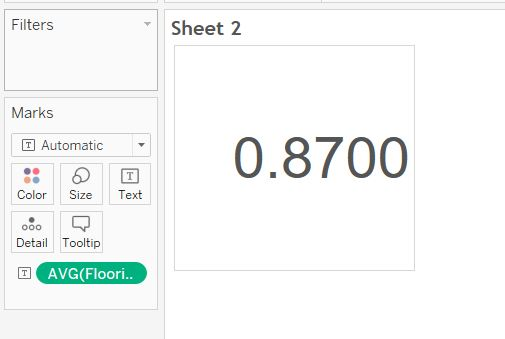## Strong-Arming Tableau: Rounding Operations

by Eliott Sacau

Forcing Tableau to round up or down at specific decimal places

Tableau has three interesting functions, ROUND() and FLOOR() and CEILING(). The ROUND() function allows you to choose the number of decimals to which you would like to round, but you cannot specify that you want a round up, or a round down, it does it based on the value at that decimal place. The FLOOR and CEILING functions offer the ability to force Tableau to round down or up, but they do not offer the ability of customising to which decimal you would like to round down or up, the default being to the nearest integer.

A quick and simple fix: within the floor function, multiply the value of the decimal you are trying to round up or down to by 10^x, where x is the number of times until the decimal we want to floor becomes the first decimal. We then wrap around the floor function a division of the same size as the previous multiplication.

It might be a bit confusing, but when we explain this, it makes more sense:

Lets say that that you have 0.872 and want to make sure that the third decimal (here 2) always get floored. This could be for a multitude of reasons, but one could be to make sure that profit, or sales are not overestimated by upward rounding. We multiply by 10^2 (100). This will force Tableau to multiply first, giving us 87.2 . We then apply the floor function at that level, leaving us with 87.We then simply divide the number by the same number that we originally multiplied it, and it returns 0.87, and voila, a simple fix to force tableau to round down at specific decimal places!Eliott Sacau

Tue 19 Dec 2017

Tue 19 Dec 2017

Fri 20 Oct 2017